## Sample Test Problems

1. A Hydrogen atom is in its 4D state. The atom decays to a lower state by emitting a photon. Find the possible photon energies that may be observed. Give your answers in eV.
The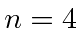state can decay into states with. (Really thestate will be suppressed due to selection rules but this is supposed to be a simple question.) The energies of the states areThe photon energy is given by the energy difference between the states.For thefinal state,eV.
For thefinal state,eV.
For the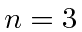final state,eV.
2. Using the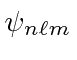notation, list all thehydrogen states. (Neglect the existence of spin.)
The states are,,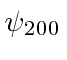,,,,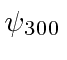,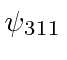,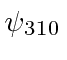,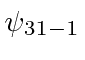,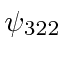,,,,.
3. Find the difference in wavelength between light emitted from thetransition in Hydrogen and light from the same transition in Deuterium. (Deuterium is an isotope of Hydrogen with a proton and a neutron in the nucleus.) Get a numerical answer.
4. An electron in the Coulomb field of a proton is in the state described by the wave function. Find the expected value of the Energy,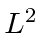and. Now find the expected value of.
5. * Write out the (normalized) hydrogen energy eigenstate.
6. Calculate the expected value of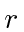in the Hydrogen state.
7. Write down the wave function of the hydrogen atom state.
8. A Hydrogen atom is in itsstate. The atom decays to a lower state by emitting a photon. Find the possible photon energies that may be observed. Give your answers in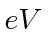.
9. A Hydrogen atom is in the state: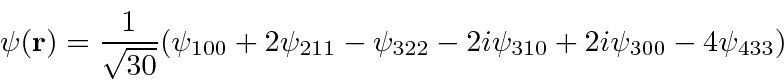For the Hydrogen eigenstates,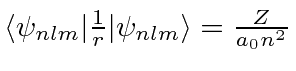. Find the expected value of the potential energy for this state. Find the expected value of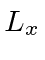.
10. A Hydrogen atom is in its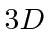state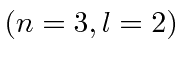. The atom decays to a lower state by emitting a photon. Find the possible photon energies that may be observed. Give your answers in.
11. The hydrogen atom is made up of a proton and an electron bound together by the Coulomb force. The electron has a mass of 0.51 MeV/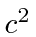. It is possible to make a hydrogen-like atom from a proton and a muon. The force binding the muon to the proton is identical to that for the electron but the muon has a mass of 106 MeV/.
a)
What is the ground state energy of muonic hydrogen (in eV).
b)
What is theBohr Radius'' of the ground state of muonic hydrogen.
12. A hydrogen atom is in the state:Find the possible measured energies and the probabilities of each. Find the expected value of.
13. Find the difference in frequency between light emitted from the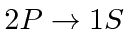transition in Hydrogen and light from the same transition in Deuterium. (Deuterium is an isotope of Hydrogen with a proton and a neutron in the nucleus.)
14. Tritium is an isotope of hydrogen having 1 proton and 2 neutrons in the nucleus. The nucleus is unstable and decays by changing one of the neutrons into a proton with the emission of a positron and a neutrino. The atomic electron is undisturbed by this decay process and therefore finds itself in exactly the same state immediately after the decay as before it. If the electron started off in the(,) state of tritium, compute the probability to find the electron in the ground state of the new atom with Z=2.
15. Ata hydrogen atom is in the state. Calculate the expected value ofas a function of time.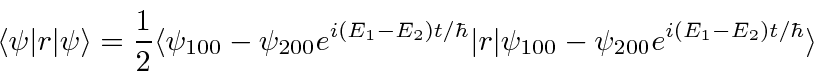The angular part of the integral can be done. All the terms of the wavefunction contain a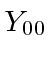anddoes not depend on angles, so the angular integral just gives 1.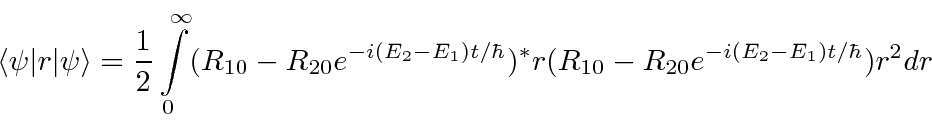The cross terms are not zero because of the.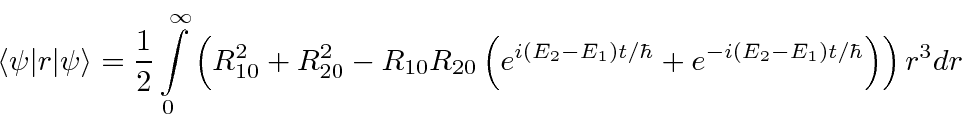Now we will need to put in the actual radial wavefunctions.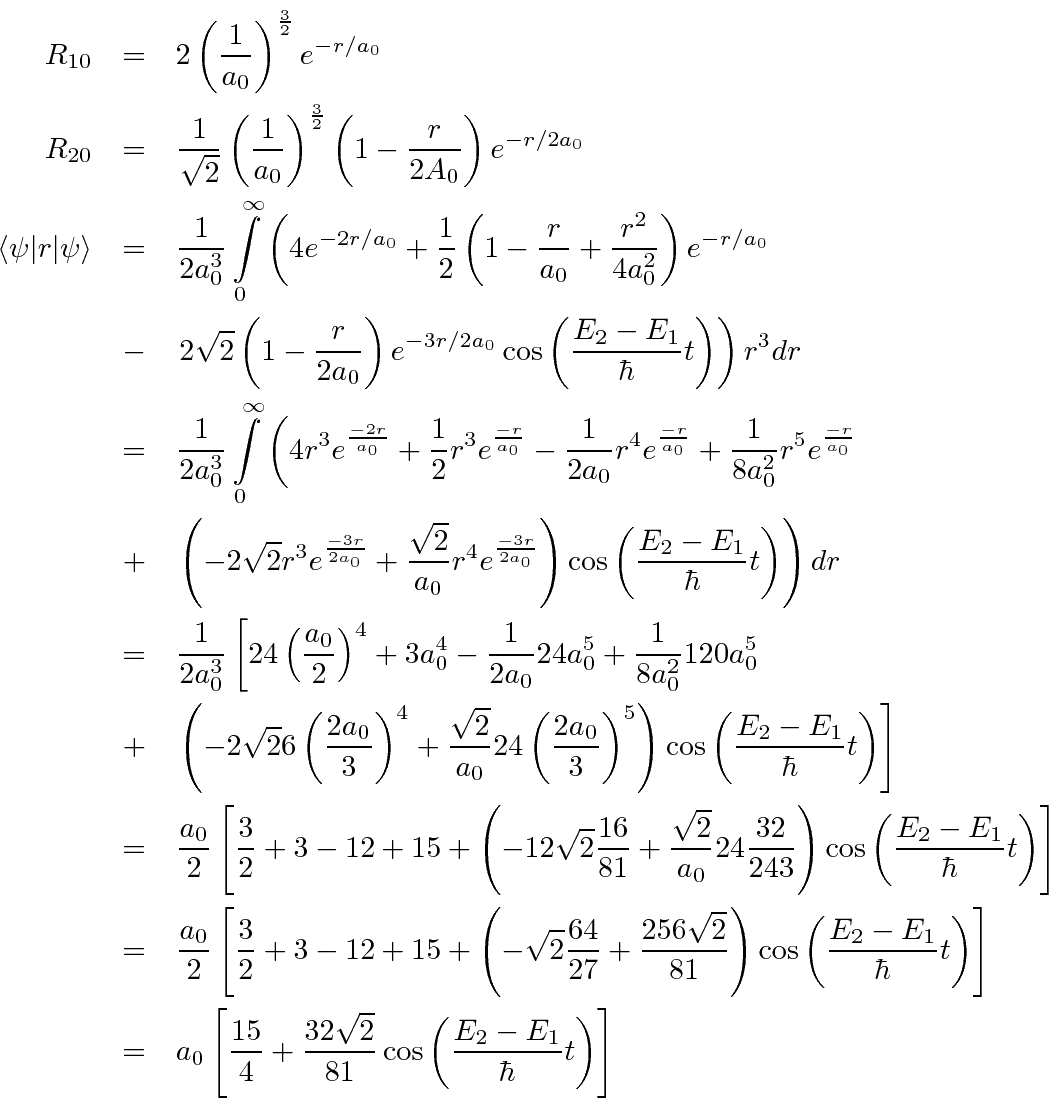Jim Branson 2013-04-22